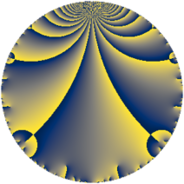# Properties

 Label 507.4.aLevel $507$ Weight $4$ Character orbit 507.a Rep. character $\chi_{507}(1,\cdot)$ Character field $\Q$ Dimension $78$ Newform subspaces $18$ Sturm bound $242$ Trace bound $4$

# Related objects

## Defining parameters

 Level: $$N$$ $$=$$ $$507 = 3 \cdot 13^{2}$$ Weight: $$k$$ $$=$$ $$4$$ Character orbit: $$[\chi]$$ $$=$$ 507.a (trivial) Character field: $$\Q$$ Newform subspaces: $$18$$ Sturm bound: $$242$$ Trace bound: $$4$$ Distinguishing $$T_p$$: $$2$$, $$5$$

## Dimensions

The following table gives the dimensions of various subspaces of $$M_{4}(\Gamma_0(507))$$.

Total New Old
Modular forms 196 78 118
Cusp forms 168 78 90
Eisenstein series 28 0 28

The following table gives the dimensions of the cuspidal new subspaces with specified eigenvalues for the Atkin-Lehner operators and the Fricke involution.

$$3$$$$13$$FrickeDim.
$$+$$$$+$$$$+$$$$20$$
$$+$$$$-$$$$-$$$$19$$
$$-$$$$+$$$$-$$$$16$$
$$-$$$$-$$$$+$$$$23$$
Plus space$$+$$$$43$$
Minus space$$-$$$$35$$

## Trace form

 $$78 q - 4 q^{2} + 320 q^{4} - 16 q^{5} - 32 q^{7} - 48 q^{8} + 702 q^{9} + O(q^{10})$$ $$78 q - 4 q^{2} + 320 q^{4} - 16 q^{5} - 32 q^{7} - 48 q^{8} + 702 q^{9} + 72 q^{10} + 96 q^{11} + 272 q^{14} + 24 q^{15} + 1384 q^{16} + 64 q^{17} - 36 q^{18} - 216 q^{19} + 92 q^{20} - 84 q^{21} + 272 q^{22} + 224 q^{23} + 180 q^{24} + 1850 q^{25} + 48 q^{28} - 544 q^{29} - 288 q^{30} - 128 q^{31} - 28 q^{32} - 192 q^{33} - 440 q^{34} - 224 q^{35} + 2880 q^{36} + 12 q^{37} - 664 q^{38} - 112 q^{40} - 296 q^{41} + 360 q^{42} + 416 q^{43} - 20 q^{44} - 144 q^{45} - 248 q^{46} + 48 q^{47} + 528 q^{48} + 4394 q^{49} + 380 q^{50} + 864 q^{51} + 600 q^{53} + 1024 q^{55} + 440 q^{56} + 84 q^{57} + 896 q^{58} + 1072 q^{59} + 1188 q^{60} - 584 q^{61} - 1068 q^{62} - 288 q^{63} + 7204 q^{64} - 1608 q^{66} + 360 q^{67} - 972 q^{68} - 168 q^{69} - 656 q^{70} + 976 q^{71} - 432 q^{72} + 1804 q^{73} - 2108 q^{74} - 480 q^{75} - 2248 q^{76} - 1704 q^{77} - 700 q^{79} + 700 q^{80} + 6318 q^{81} - 3948 q^{82} + 3072 q^{83} - 624 q^{84} - 2432 q^{85} - 2640 q^{86} + 1476 q^{87} - 1272 q^{88} - 1376 q^{89} + 648 q^{90} - 1156 q^{92} - 1428 q^{93} + 824 q^{94} + 4944 q^{95} - 840 q^{96} - 2820 q^{97} + 5644 q^{98} + 864 q^{99} + O(q^{100})$$

## Decomposition of $$S_{4}^{\mathrm{new}}(\Gamma_0(507))$$ into newform subspaces

Label Dim $A$ Field CM Traces A-L signs $q$-expansion
$a_{2}$ $a_{3}$ $a_{5}$ $a_{7}$ 3 13
507.4.a.a $1$ $29.914$ $$\Q$$ None $$-3$$ $$3$$ $$9$$ $$-2$$ $-$ $+$ $$q-3q^{2}+3q^{3}+q^{4}+9q^{5}-9q^{6}+\cdots$$
507.4.a.b $1$ $29.914$ $$\Q$$ None $$-1$$ $$3$$ $$7$$ $$-10$$ $-$ $+$ $$q-q^{2}+3q^{3}-7q^{4}+7q^{5}-3q^{6}+\cdots$$
507.4.a.c $1$ $29.914$ $$\Q$$ None $$0$$ $$-3$$ $$12$$ $$-2$$ $+$ $+$ $$q-3q^{3}-8q^{4}+12q^{5}-2q^{7}+9q^{9}+\cdots$$
507.4.a.d $1$ $29.914$ $$\Q$$ None $$1$$ $$3$$ $$-7$$ $$10$$ $-$ $+$ $$q+q^{2}+3q^{3}-7q^{4}-7q^{5}+3q^{6}+\cdots$$
507.4.a.e $1$ $29.914$ $$\Q$$ None $$3$$ $$3$$ $$-9$$ $$2$$ $-$ $+$ $$q+3q^{2}+3q^{3}+q^{4}-9q^{5}+9q^{6}+\cdots$$
507.4.a.f $2$ $29.914$ $$\Q(\sqrt{14})$$ None $$-2$$ $$-6$$ $$-24$$ $$0$$ $+$ $+$ $$q+(-1+\beta )q^{2}-3q^{3}+(7-2\beta )q^{4}+\cdots$$
507.4.a.g $2$ $29.914$ $$\Q(\sqrt{3})$$ None $$0$$ $$-6$$ $$0$$ $$0$$ $+$ $-$ $$q-3q^{3}-8q^{4}+3\beta q^{5}+6\beta q^{7}+9q^{9}+\cdots$$
507.4.a.h $3$ $29.914$ 3.3.3144.1 None $$-2$$ $$9$$ $$-4$$ $$-30$$ $-$ $+$ $$q+(-1+\beta _{1})q^{2}+3q^{3}+(3+\beta _{2})q^{4}+\cdots$$
507.4.a.i $4$ $29.914$ $$\mathbb{Q}[x]/(x^{4} - \cdots)$$ None $$-2$$ $$-12$$ $$6$$ $$14$$ $+$ $+$ $$q-\beta _{1}q^{2}-3q^{3}+(5+\beta _{1}+\beta _{2})q^{4}+\cdots$$
507.4.a.j $4$ $29.914$ 4.4.5054412.1 None $$0$$ $$-12$$ $$0$$ $$0$$ $+$ $-$ $$q+\beta _{1}q^{2}-3q^{3}+(7+\beta _{3})q^{4}-\beta _{2}q^{5}+\cdots$$
507.4.a.k $4$ $29.914$ $$\Q(\sqrt{3}, \sqrt{17})$$ None $$0$$ $$-12$$ $$0$$ $$0$$ $+$ $-$ $$q+\beta _{3}q^{2}-3q^{3}+9q^{4}+(3\beta _{1}-2\beta _{3})q^{5}+\cdots$$
507.4.a.l $4$ $29.914$ 4.4.1362828.1 None $$0$$ $$12$$ $$0$$ $$0$$ $-$ $-$ $$q+\beta _{1}q^{2}+3q^{3}+(4+\beta _{3})q^{4}+(2\beta _{1}+\cdots)q^{5}+\cdots$$
507.4.a.m $4$ $29.914$ $$\mathbb{Q}[x]/(x^{4} - \cdots)$$ None $$2$$ $$-12$$ $$-6$$ $$-14$$ $+$ $+$ $$q+\beta _{1}q^{2}-3q^{3}+(5+\beta _{1}+\beta _{2})q^{4}+\cdots$$
507.4.a.n $9$ $29.914$ $$\mathbb{Q}[x]/(x^{9} - \cdots)$$ None $$-8$$ $$-27$$ $$-41$$ $$-1$$ $+$ $-$ $$q+(-1+\beta _{1})q^{2}-3q^{3}+(3-2\beta _{1}+\beta _{2}+\cdots)q^{4}+\cdots$$
507.4.a.o $9$ $29.914$ $$\mathbb{Q}[x]/(x^{9} - \cdots)$$ None $$-6$$ $$27$$ $$-33$$ $$-83$$ $-$ $+$ $$q+(-1-\beta _{3})q^{2}+3q^{3}+(5+\beta _{3}+\beta _{5}+\cdots)q^{4}+\cdots$$
507.4.a.p $9$ $29.914$ $$\mathbb{Q}[x]/(x^{9} - \cdots)$$ None $$6$$ $$27$$ $$33$$ $$83$$ $-$ $-$ $$q+(1+\beta _{3})q^{2}+3q^{3}+(5+\beta _{3}+\beta _{5}+\cdots)q^{4}+\cdots$$
507.4.a.q $9$ $29.914$ $$\mathbb{Q}[x]/(x^{9} - \cdots)$$ None $$8$$ $$-27$$ $$41$$ $$1$$ $+$ $+$ $$q+(1-\beta _{1})q^{2}-3q^{3}+(3-2\beta _{1}+\beta _{2}+\cdots)q^{4}+\cdots$$
507.4.a.r $10$ $29.914$ $$\mathbb{Q}[x]/(x^{10} - \cdots)$$ None $$0$$ $$30$$ $$0$$ $$0$$ $-$ $-$ $$q+\beta _{1}q^{2}+3q^{3}+(6+\beta _{4})q^{4}+(\beta _{1}+\beta _{2}+\cdots)q^{5}+\cdots$$

## Decomposition of $$S_{4}^{\mathrm{old}}(\Gamma_0(507))$$ into lower level spaces

$$S_{4}^{\mathrm{old}}(\Gamma_0(507)) \cong$$ $$S_{4}^{\mathrm{new}}(\Gamma_0(13))$$$$^{\oplus 4}$$$$\oplus$$$$S_{4}^{\mathrm{new}}(\Gamma_0(39))$$$$^{\oplus 2}$$$$\oplus$$$$S_{4}^{\mathrm{new}}(\Gamma_0(169))$$$$^{\oplus 2}$$